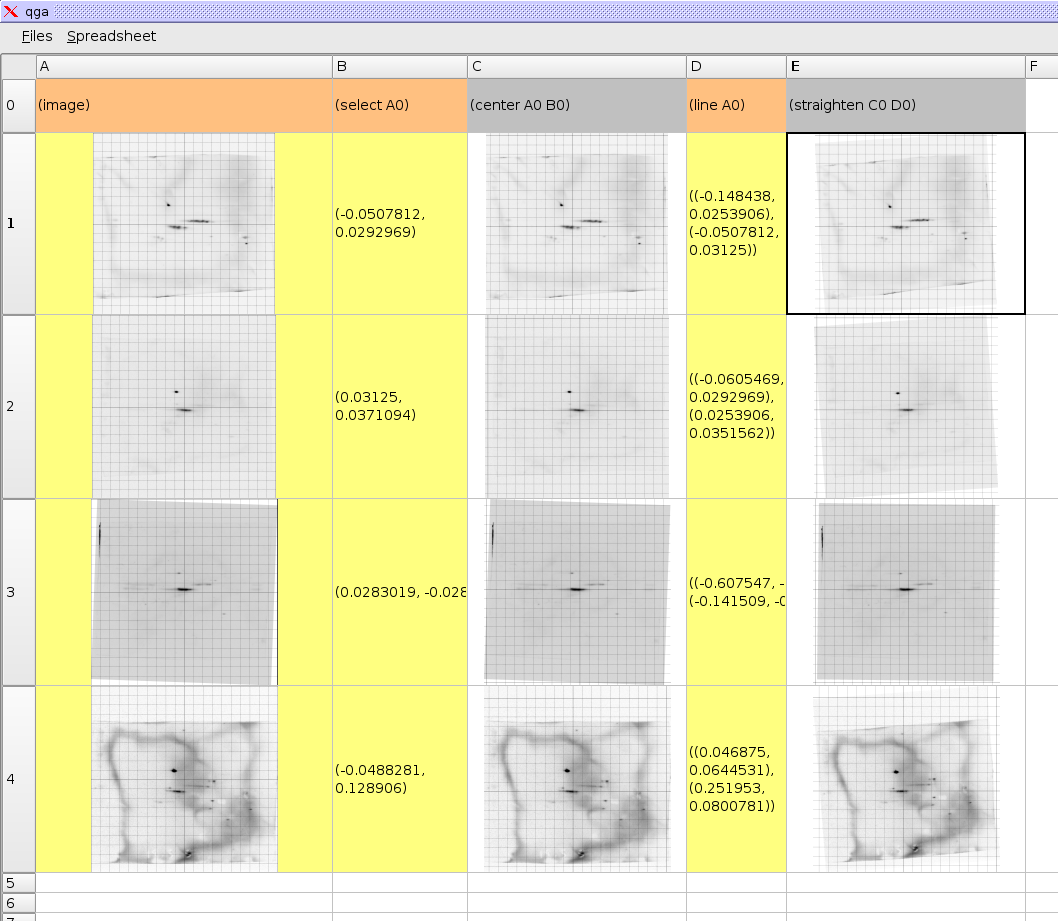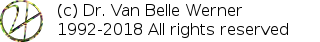Project Home

# Step 3: Rotating 2DE Images

Explains how to rotate images

`(line A0)(straighten C0 D0) `

With 2DE images one often needs to rotate the images such that the horizontal axis is correct with the direction of the gel. In the IIS we can do this by adding a formula that allows us to select a line and then straighten out the image using the angle of this line. We do this by adding in cell D0 the formula (line A0), which allows us to select a line on the image using two coordinates. In formula E0 we add (straighten C0 D0), which will rotate image C0 to the nearest 0° or 90° angle.http://werner.yellowcouch.org/mailto:werner@yellowcouch.org